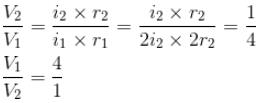Courses

# Magnetostatics MCQ Level - 2

## 10 Questions MCQ Test Electricity & Magnetism | Magnetostatics MCQ Level - 2

Description
This mock test of Magnetostatics MCQ Level - 2 for IIT JAM helps you for every IIT JAM entrance exam. This contains 10 Multiple Choice Questions for IIT JAM Magnetostatics MCQ Level - 2 (mcq) to study with solutions a complete question bank. The solved questions answers in this Magnetostatics MCQ Level - 2 quiz give you a good mix of easy questions and tough questions. IIT JAM students definitely take this Magnetostatics MCQ Level - 2 exercise for a better result in the exam. You can find other Magnetostatics MCQ Level - 2 extra questions, long questions & short questions for IIT JAM on EduRev as well by searching above.
QUESTION: 1

### Figure here shows three close in all cases the circular path has radius r and straight ones are infinitely long. For same current the magnetic field at the centre P in cases 1, 2, 3 have the ratio :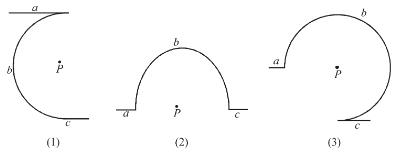Solution:

For first figure magnetic field at O  due to straight part (a)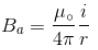Magnetic field at O  due to circular part (b)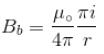Magnetic field at O  due to straight part (c)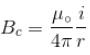So, net magnetic field at the centre of first figure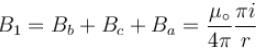For second figure

Magnetic field at O  due to two straight and one circular path is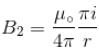For third figure magnetic field at O  due to straight part (a)
Ba = 0
Magnetic field at O  due to circular part (b)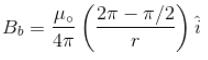Magnetic field at C due to straight part  (c)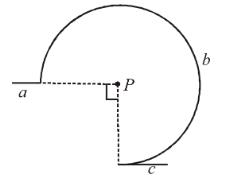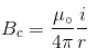So net magnetic field at centre of third figure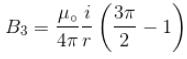From equation (i), (ii) and (iii)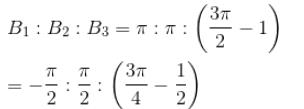The correct answer is: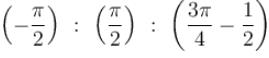QUESTION: 2

### A disc of radius R rotates constant angular velocity ω about its own axis surface density of this disc varies as σ = αr2, where r is the distance from the centre of the disc. Determine the magnetic field  intensity at the centre of disc.

Solution:

To find the magnetic field due to charge on rotating disc. Consider a ring of radius r and thickness dr.

So, dA = 2πrdr

Charge on element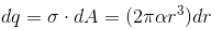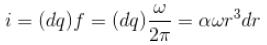Magnetic field at the centre due to charge element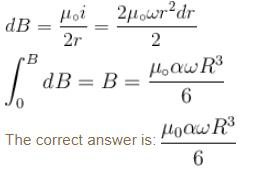QUESTION: 3

### For a solenoid of length l, having N turns, the magnetic field at a point on its axis is given by :

Solution:

Let a solenoid of length l, having N  turns, is given as figure. To find magnetic field at a point on its axis, consider an element of a solenoid in the form of ring of radius a (same as solenoid) and thickness dx.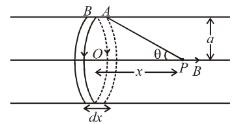Let n  be the number of turns per unit length.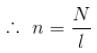Number of turns in dx length = dN = ndx
Let I  be the current flowing in the soil.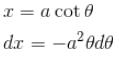Using result of the ring, the magnetic field at the point P  is given by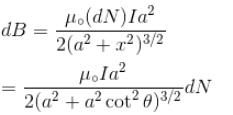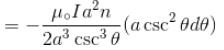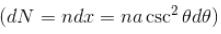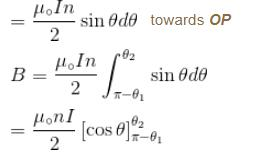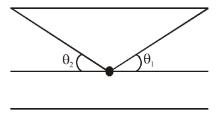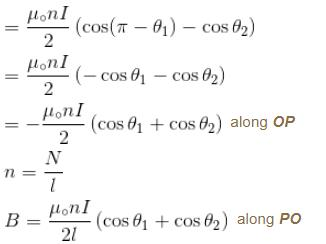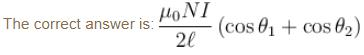QUESTION: 4

A particle of mass m and charge q, moving with velocity v enters region II normal to the boundary as shown in the figure. Region II has a uniform Magnetic field perpendicular to the plane of he paper. The length of the Region II is l. Choose the incorrect choice(s)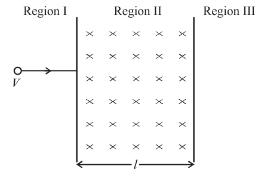Solution:

In region II,

Magnetic field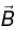is perpendicular to velocity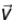therefore, path of particle is circle is region II Particle enters in region III if, radius of circular path,

r > l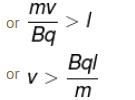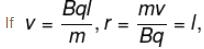particle will turn back and path length will be maximum. If particle returns to region I, time spent in region II will be :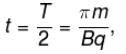which is independent of V
The correct answer is: The particle enter Region III only if its velocity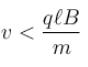QUESTION: 5

The magnetic field at the centre of square of side a is :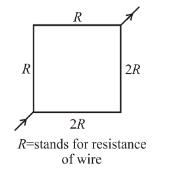Solution:

Magnetic field due to a current carrying conductor at a point on the perpendicular axis passing through mid point is given by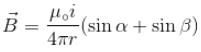α and β are the angle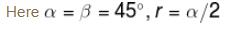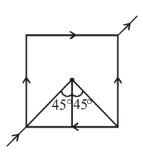So,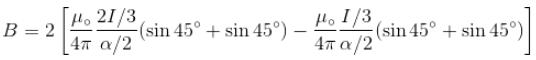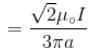The correct answer is: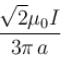QUESTION: 6

A coil of 100 turns and area 2 × 10–2 m2 is pivoted about a vertical diameter in a uniform magnetic field and carries a current of 5A. When the coil is held with its plane in north south direction it experiences a couple of 0.33 Nm. When the plane is east-west, the corresponding couple is 0.4 Nm the value of magnetic induction is [Neglect earth’s magnetic field]

Solution:

Torque due to magnetic field,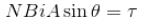NBi  and  A  are constant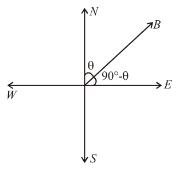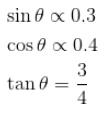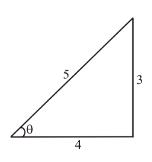From equation (i)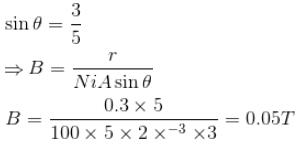The correct answer is: 0.05 T

QUESTION: 7

A wire of length L is bent in the form of a circular coil and current i is passed through it. If this coil is placed in a magnetic field then the torque acting on the coil will be maximum when the number of turns is :

Solution:

The are n  turns having i  current each, so  I = ni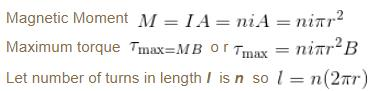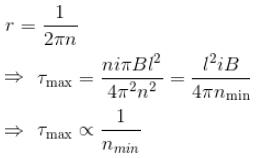nmin = 1

QUESTION: 8

Which of the following statement is (are) correct in the given figure?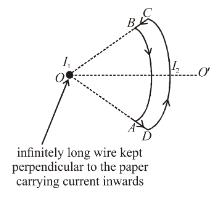Solution:

We know that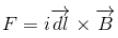In case of wire BA :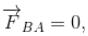because magnetic line are parallel to this wire.
In case of wire CD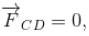because magnetic lines are anti parallel to this wire.

In case of  CB, and  AD,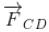is perpendicular to paper outward and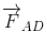is perpendicular to paper inward. These two force (although calculated by integration) cancel each other but produce a torque which tend to relate the loop in clockwise direction about an axis OO'.
The correct answer is: loop will rotate clockwise about axis OO'  when seen from O

QUESTION: 9

A charge q is moving with a velocity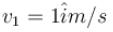at a point in a magnetic field and experience a force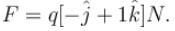If the charge is moving with velocityat the same point, it experience a force

F2 = q(i - k)N   The magnetic induction B at that point is :

Solution:

Let the magnetic field is,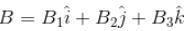Applying  Fm = q(V × B)  two times, we have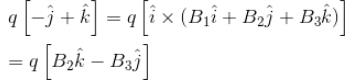Comparing two sides, we get B2 = 1 and B3 = 1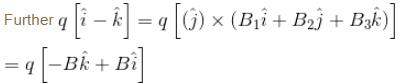Again comparing we get, B1 = 1 and B3 = 1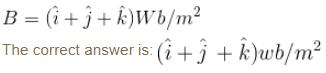QUESTION: 10

Two circular coil 1 and 2 are made from the same wire but the radius of the 1st coil is twice that of the 2nd coil. What is the ratio of potential difference applied across them, so that magnetic field at their centre is same ?

Solution:

Magnetic field due to current in coil-1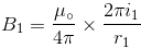Magnetic field due to current in coil-2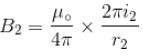But  B1 = B2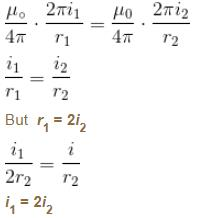Now, ratio of potential difference